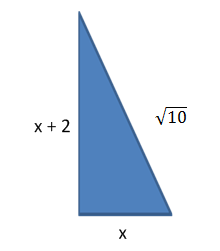### Sample Problem

Use the Pythagorean Theorem to find the unknown lengthsx =

#### Solution

Based on the pythagorean theorem we know:

x2 + (x + 2)2 =x2 + x2 + 4x + 4 = 10

2x2 + 4x – 6 = 0

2(x – 1)(x + 3) = 0

x = 1  and  x = -3

Since lengths must be positive, x = 1